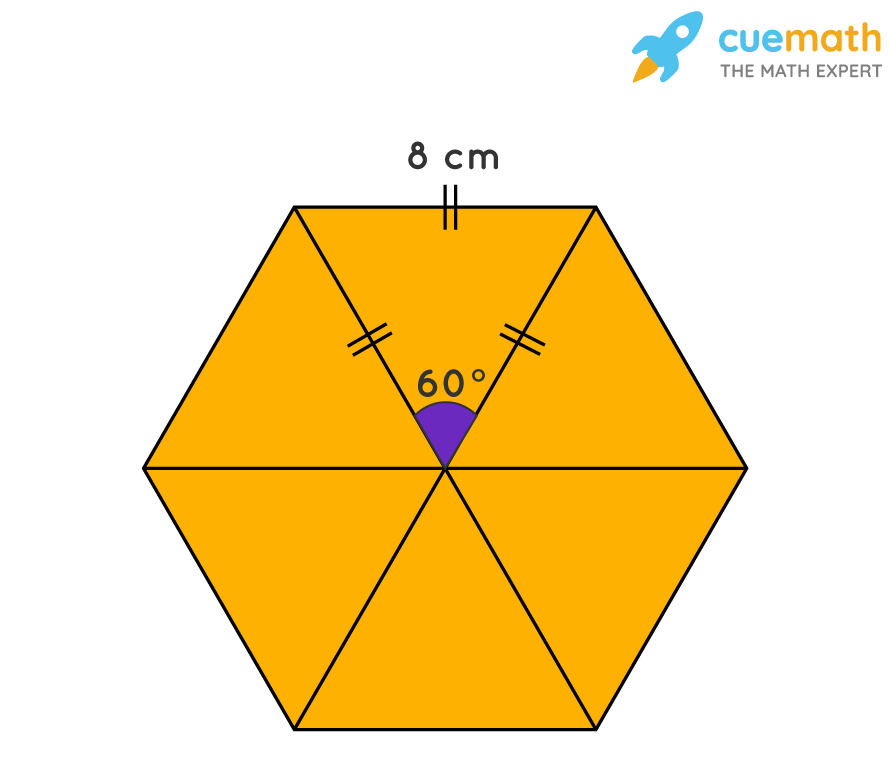# Each Side of the Regular Hexagon Below Measures 8 Cm. What Is the Area of the Hexagon?

## Question: Each side of the regular hexagon below measures 8 cm. What is the area of the hexagon?

A regular hexagon is a polygon with 6 equal sides and equal angles

## Explanation:

An equilateral triangle is a regular polygon with 3 equal sides with each angle equal to 60°Area of regular hexagon = 6 × area of an equilateral triangle

⇒ Area of regular hexagon = 6 × (√3 / 4) × a2 , where a is the side of the equilateral triangle.

Here a = 8 cm

⇒ Area of regular hexagon = 6 × (√3 / 4) × 82

⇒ Area of regular hexagon = 6 × (√3 / 4) × 64

⇒ Area of regular hexagon = (6 × 64 × √3 )/ 4

⇒ Area of regular hexagon = (6 × 16 × √3 )

⇒ Area of regular hexagon = 96√3  square centimeters.